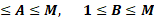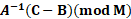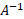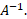The Affine cipher is a type of monoalphabetic substitution cipher where each letter in an alphabet is mapped to its numeric equivalent, encrypted using a simple mathematical function, and then converted back to a letter.

## The algorithm

The “key” for the Affine cipher consists of 2 numbers, A and B. In this example we are going to use a 26-character alphabet (M = 26).

A should be chosen to be relatively prime to M (A should have no factors in common with M).

For example, 15 and 26 have no factors in common, so, 15 is an acceptable value for A.

However, 12 and 26 have factors in common (that is 2), so, 12 cannot be used for a value of A. When encrypting, we first convert all the letters to numbers (a=0, b=1…. z=25).

The ciphertext letter C for any given letter P is (P is the number representing a letter).

C = AP + B (mod M), 1≤A≤M,       1≤B≤MThe decryption function is:

P = A-1(C-B)(mod M)Where A-1is the multiplicative inverse of A in the group of integers modulo M.

To find a multiplicative inverse, we need to find a number X such that:

AX = 1 (mod M)

If we find the number X such that the equation is true, then X is the inverse of A, and we call it A-1.The easiest way to solve this equation is to search each of the numbers 1 to 25, and see which one satisfies the equation.

If you now multiply X and A and reduce the result (mod 26), you will get the answer 1. Remember, this is just the definition of an inverse i.e. if A*X = 1 (mod 26), then X is an inverse of A (and A is an inverse of X).

We now use the value of X we calculated as A-1. This allows us to perform the decryption step.

As stated above, M does not have to be 26, it is simply the number of characters in the alphabet you choose to use.

## Example

Encryption uses a classic alphabet, and two integers, called coefficients or keys A and B, these are the parameters of the affine function Ax + B.

## How to encrypt using affine cipher?

For example, to encrypt “KIFANGA” with the alphabet “ABCDEFGHIJKLMNOPQRSTUVWXYZ” and keys A = 5, B = 3.

For each letter of the alphabet is associated to the value of its position in the alphabet (start at 0).

For example, A = 0, B = 1, …Z = 25. It is possible to use A = 1… Y =25, Z = 0 with the alphabet “ZABCDEFGHIJKLMNOPQRSTUVWXY” (not recommended).

For each letter of value x of the plain text, is associated a value y, resulting of the affine function y = A*x + B mod 26 (with 26 the alphabet size).

For each value y, corresponds a letter with the same position in the alphabet, it is the encoded letter. The Affine ciphertext is the replacement of the letters by the new ones.

For example, “KIFANGA” is encrypted as “BRCDQHD”.

 Plain letter x Y (mod 26) Encoded letter K 10 5*10+3=53=1 B I 8 5*8+3=43=17 R

## How to decrypt affine cipher?

Affine decryption requires to know the two keys A and B and the alphabet.

For example, let’s decrypt the message “BRCDQHD” with keys A=5 and B=3.

For each letter of the alphabet corresponds the value of its position in the alphabet.

For example, the alphabet “ABCDEFGHIJKLMNOPQRSTUVWXYZ”, starting at 0 gives A=0, B=1, …, Z=25. There is also A=1, …, Y=25 and Z=0 with the alphabet “ZABCDEFGHIJKLMNOPQRSTUVWXY”.

For each letter of value y of the message, corresponds a value x, result of the inverse function x = A’*(y-B) mod 26.

The value A’ is an integer such as A*A’=1 mod 26.

To find A’, calculate a modular inverse.

For example, a coefficient A’ for A=5 with an alphabet size of 26 is 21 because:

5*21=85=1 mod 26

For letter B (y=1),

X =A’*(1-B) = 21*(1-3) = -42 mod 26 =10

For each value x, corresponds a letter with the same position in the alphabet: the coded letter. The plaintext is the replacement of all characters with calculated new letters.

For example, for letter B (x=10) corresponds the letter at position 10: K, etc.

The original plain text is “KIFANGA”.

## A JavaScript Example

Use the above Affine cipher decoder and encoder to encrypt/decrypt simple messages.

## How to recognize an affine ciphertext?

A message encrypted by Affine has a coincidence index close to the plain text language’s one. Any reference to an affine function (in a straight line), a graph, an abscissa or an ordinate is a clue.

## How to decipher affine without coefficient A and B?

To crack Affine, it is possible to brute force all values for A and B coefficients.

If the alphabet is 26 characters long, then A coefficient has only 12 possible values, and B has 26 values, so there are only 312 tests to try.

## What is the problem with using the key a=4 and b=5?

This key creates the situation where more than one plaintext letter is encrypted to the same ciphertext letter.

This means that when it comes to decrypting, the recipient will be unable to know which one of the plaintext letters has been used.

Clearly this is huge problem in using the Affine cipher, and it is essential for the key to be chosen carefully.

## Can you find other keys that have the same problem?

This problem occurs since the multiplicative inverse of A does not exist module M. That is, there is more than one number that can be multiplied by 4 to get 1 modulo 26.

There is a quick way to check if an inverse exists for a given M and A (relying on an advanced mathematics topic called group theory).

The inverse of A modulo M exists if and only if A and M are coprime (that is they have no common factor other than 1).

Hence, A = 4 does not work when M = 26, as they have a common factor of 2 (2 goes into both 4 and 26), but A = 5 does work, since 5 and 26 are coprime.

We can also calculate all the possible keys for the Affine cipher.

Since, for the standard alphabet, there are 12 numbers less than 26 which are coprime to 26, and for each of these there are 26 possibilities for the value of B, we have a total of 12 *26 = 312 possible keys for the Affine cipher.

Due to this relatively low number of possible keys, the Affine cipher is once again susceptible to a brute force attack, especially in the age of computers, and hence not a very secure cipher.

Given this, we can also make the cipher a bit more secure by choosing an alphabet with a prime number of elements (since then all the numbers less than our prime are coprime to it, by definition).

Thus, with our alphabet of 31 elements (the 26 letters, space and 4 punctuation marks), we have 30 possible values for A, and still 26 values for B each time, and hence there are 30 * 26 = 780 possible keys for the alphabet.

Although this is significantly more secure keys than the standard alphabet, with computing power we can still perform a brute force attack within a few minutes.

One other way to adapt the Affine cipher quite simply is to change the numbering of the letters. The simplest way to do this is to label A as 1, B as 2 and so on.

Although this is more intuitive, the math in the other version is easier and has a lot of advantages.

Using this will clearly give different results compared to the normal way of assigning values to letters, but the process is identical.

## How to compute A’ value?

Calculate the modular inverse of A, modulo the length of the alphabet.

## How to compute B’ value?

B’ has the same value as B.

## What is the A’ values?

The value of A’ depends on A but also on the alphabet’s length, if it is a classic one, it is 26 characters long. The values of A’ are then:

 A = 1 A’ = 1 A = 3 A’ = 9 A = 5 A’ = 21 A = 7 A’ = 15 A = 9 A’ = 3 A = 11 A’ = 19 A = 15 A’ = 7 A = 17 A’ = 23 A = 19 A’ = 11 A = 21 A’ = 5 A = 23 A’ = 17 A = 25 A’ = 25

## Why is there a constraint on the value of A?

The Bezout’s theorem indicates that A’ only exists if A and 26 (alphabet length) are coprime. This limits A values to 1, 3, 5, 7, 9, 11, 15, 17, 19, 21, 23 and 25 (if the alphabet is 26 characters long).

## Is it possible to use a key A not coprime with 26?

Yes, but an automatic decryption process becomes impossible, a single encoded letter will have multiple plain letters possible.

## Does a negative value for A exists?

Yes, but it exists a positive corresponding value, a value of A = -1 is equals to a value of A = 25 (because 25 = -1 mod 26).

## Is there a limitation on B value?

No, B can take any value. All the values of B modulo 26 (length of the alphabet) are equivalent. So, if B is negative, there is an equivalent positive value of B.

## Why is this encryption so called affine?

In mathematics, an affine function is defined by addition and multiplication of the variable (often x) and written f(x) = ax + b.

The affine cipher is similar to the f function as it uses the values a and b as a coefficient and the variable x is the letter to be encrypted.

Recommended Tools